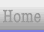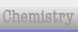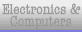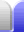# Sequences Overview

### Notation

In mathematics, a sequence is a set of sequentially ordered numbers or objects. They are often related by a rule from which a particular term might be calculated from previous terms, or from its position in the sequence.

The simplest sequence is the natural numbers, where each number is the same as its position in the sequence. We can write that as a "rule" by assigning "n" to its position. Then the "n"th number will be xn.

1, 2, 3, 4, 5, 6, ...

xn = n

We can also write a rule for calculating the value of each number from the value of the immediately preceeding number (the one just before it). In the case of natural numbers:

xn+1 = xn + 1

### Square numbers

 Square numbers are numbers which are the result of a smaller whole number multiplied by itself. 9 is a square number made by multiplying 3 by itself: 3 x 3 = 9. If you have a square number of small square tiles you can arrange them into a larger square. Let's look at the sequence of square numbers and its rules. 1, 4, 9, 16, 25, 36, 49, 64, 81, 100, ... xn = n2 xn+1 = ...Trying to find a rule for finding xn+1 the first thing we notice is that the differences between consecutive numbers itself forms a simple sequence. 1, 3, 5, 7, 9, 11, ... yn = 2n – 1 yn+1 = yn + 2 ∴ yn+1 = (2n – 1) + 2 yn+1 = 2n + 1 So now we know the difference between consecutive numbers, and we can put that into the equation for our square numbers sequence. 1, 4, 9, 16, 25, 36, 49, 64, 81, 100, ... xn = n2 xn+1 = xn + 2n + 1 ∴ xn+1 = n2 + 2n + 1 Square trivia: Squares of even numbers are even, for example 6 x 6 = 36. Squares of odd numbers are odd. For example, 7 x 7 = 49. A square number can only end with digits 00, 1, 4, 6, 9, or 25. The square of n is the sum of all odd numbers less than 2n. The sum of any two consecutive triangular numbers is a square number. (Triangular numbers – a very interesting sequence – can be arranged in a triangle: 1, 3, 6, 10, 15, 21, ...) When an object falls (ignoring air resistance) the distance it travels in each fixed amount of time follows the square numbers. See the picture at right.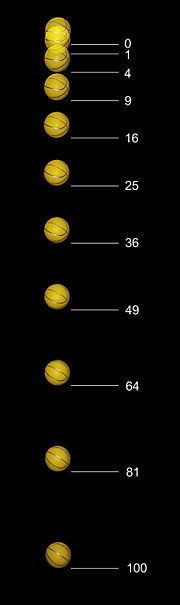### Cubic numbers

Cubic numbers are those which result from multiplying a whole number by itself then by itself again. For example, 8 is a cubic number made from 2 x 2 x 2. If you have a cubic number of small cubes you can stack them together to make a larger cube.

1, 8, 27, 64, 125, 216, ...

xn = n3

For example, if a Rubik's Cube was made of individual small cubes stacked together, there would be 27 small cubes. 3 x 3 x 3 = 27.

The 6th cubic number, 216, is the reason why small spherical magnets are sold in quantities of 216 – so they can be grouped into a 6 x 6 x 6 cube. (Small spherical magnets are now illegal to buy in or import into New Zealand.)Home Astronomy Chemistry Electronics & Computers Mathematics Physics Field Trips Turn on javascript for email link